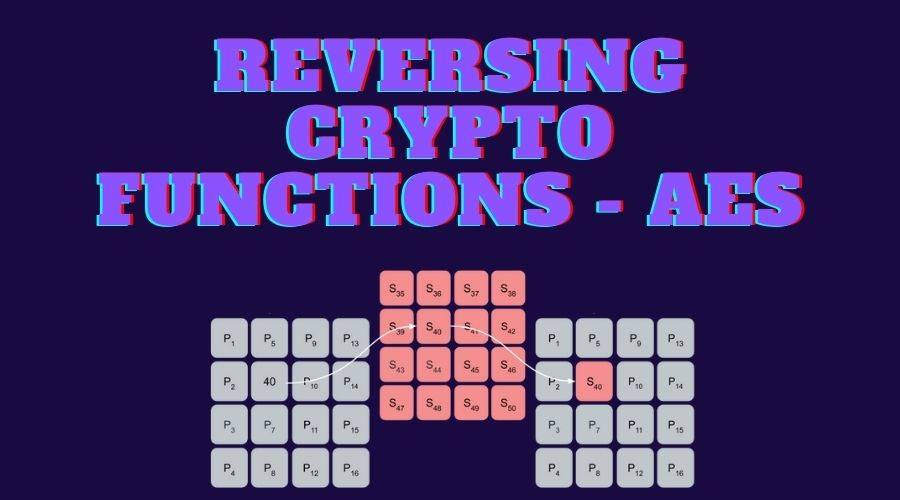# Reverse Engineering Crypto Functions: AES

### 12 December 2021

By Jacob Pimental

The Advanced Encryption Standard (AES) algorithm is a successor to the Data Encryption Standard (DES). With the advancement of technology, the key length and small block size of DES made it less secure. In 1997, NIST announced a competition to come up with a stronger algorithm; thus, AES was born.

This post is a follow-up to my previous post on reverse engineering cryptographic algorithms where I provided information on the RC4 and Salsa20 algorithms. In this post, I will explain how the AES algorithm works and how you can identify it when reverse engineering an application.

1. Basic AES Encryption
2. AES Lookup Table (T-Table) Method
3. Identifying AES in Assembly Code
1. Identifying the S-Box/T-Tables
2. Identifying Normal AES Functions
3. Identifying Key Expansion
4. Conclusion

## Basic AES Encryption

The AES Algorithm will break up a plaintext string into 16 byte “blocks” that undergo several rounds of encryption. The steps are detailed in the official AES documentation:

1. Expand provided key (Key Expansion Section)
2. AddRoundKey function on the plaintext block using the expanded key
3. Multiple encryption Rounds
1. SubBytes function on block
2. ShiftRows function on block
3. MixColumns function on block
4. Final SubBytes call
5. Final ShiftRows call

The number of rounds that are used and the size of the expanded key, `EK`, are dependent on the size of the provided key. The following table shows the correlation between the key length, the number of rounds, and the length of the expanded key:

Key Length (Bits) Number of Rounds Expanded Key Length (Words)
128 10 44
192 12 72
256 14 112

Example code for AES might look like the following:

``````def cipher(self, pt):
"""Basic AES implementation.

Args:
pt (bytes): Plaintext to encrypt

Returns:
list: Encrypted Ciphertext formatted as list of bytes
"""
state = self.rows_to_columns(pt)
self.add_round_key(state, self.ek[0:4]) #ek is the expanded key
for round in range(1, 10):
self.sub_bytes(state)
self.shift_rows(state)
self.mix_columns(state)
self.sub_bytes(state)
self.shift_rows(state)
return state
``````

### State

The state for the traditional AES algorithm is depicted as a 4x4 matrix to which the plaintext block is mapped. The block is copied to this matrix row by row; meaning that the first byte will be in column one, row one and the second byte will be in column one, row two. This can be seen in the following image where `P` denotes the plaintext array and `Px` denotes the byte at index `x` of the plaintext array: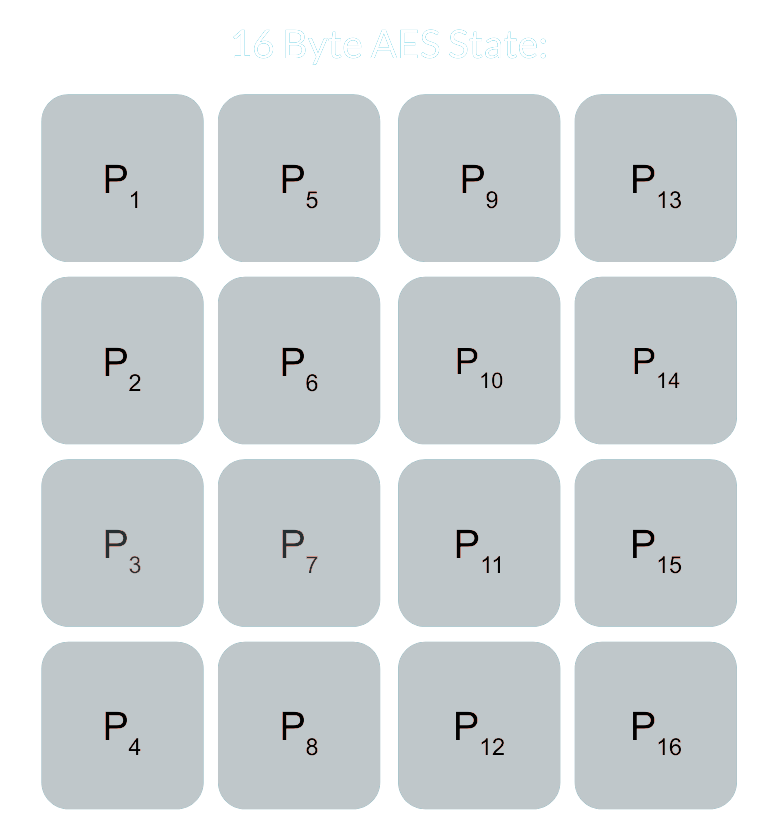Basic AES State

### Key Expansion

The expanded key for AES is a list of four-byte words that are derived from the original key. The first words in the array are the same as the original key. For example, the first four bytes in the key array would be the first word in the expanded key, `EK`, like so:

``````key = [0x00, 0x11, 0x22, 0x33, 0x44, 0x55, 0x66, 0x77, 0x88, 0x99, 0xaa, 0xbb, 0xcc, 0xdd, 0xee, 0xff]

expanded_key = [0x00112233,
0x44556677,
0x8899aabb,
0xccddeeff,
...]
``````

Each additional word is the result of XORing the previous word by the word at index `i - NK` where `i` is the current index and `NK` is the number of four-byte words that are in the original cipher key (4, 6, or 8 depending on the number of bits in the key). When the current index is a multiple of `NK`, the previous word is transformed before undergoing the XOR. This consists of the word being rotated to the left by one (i.e `0xAABBCCDD` becomes `0xBBCCDDAA`), then each byte being substituted by a value in AES’s Substitution Box (S-Box)– a 256 byte array. This new four-byte word is then XORed by a “round constant”, `RCON`. The round constant, `RCON`, can be computed from the algorithm: `2i-1 << 24` which, however, uses a Galois Field to perform multiplication instead of normal Base-10 multiplication. This type of multiplication is beyond the scope of this article, but you can read more about it here. The round constants can also be hardcoded in with the following values for the standard AES-128 algorithm:

Index 0 1 2 3 4 5 6 7 8 9 10
Values None 0x01 0x02 0x04 0x08 0x10 0x20 0x40 0x80 0x1B 0x36

The entire AES-128 key expansion algorithm could look like the following Python code:

``````# Round Constants
RCON = [None, 0x01, 0x02, 0x04, 0x08, 0x10, 0x20, 0x40, 0x80, 0x1B, 0x36]

def subword(self, word):
"""AES SubWord Method.

Args:
word (list): Word to transform

Returns:
int: Transformed word
"""
bs = struct.pack('>I', word)
nw = []
for b in bs:
nw.append(self.SBOX[b])
nw = struct.unpack('>I', bytes(nw))
return nw

def rotword(self, word):
"""AES RotateWord Method.

Args:
word (list): Word to transform

Returns:
int: Transformed word
"""
bs = list(struct.pack('>I', word))
bs.append(bs.pop(0))
nw = struct.unpack('>I', bytes(bs))
return nw

def key_expansion(self, key):
"""AES Key Expansion Algorithm.

Args:
key (bytes): Key to expand

Returns:
list: List of words for expanded key
"""
ek = []
i = 0
while i < 4:
b = bytes(key[i*4:(i*4)+4])
w = struct.unpack('>I', b)
ek.append(w)
i += 1
while i < 44: # 44 is the number of words in the expanded key
tmp = ek[i-1]
if i % 4 == 0: # Hardcoding the NK value "4" to be AES-128 specific
rcon_val = struct.unpack('>I',
bytes([self.RCON[i//4], 0, 0, 0]))
tmp = self.subword(self.rotword(tmp)) ^ rcon_val
nw = tmp ^ ek[i-4]
ek.append(nw)
i += 1
return ek
``````

### Substitution Box (S-Box)

The AES S-Box is a 256-byte hardcoded array. This array is used for the SubWord function in the Key Expansion algorithm, as well as the SubByte function in the main cipher. The bytes in the S-Box are as follows:

0 1 2 3 4 5 6 7 8 9 A B C D E F
0x63 0x7C 0x77 0x7B 0xF2 0x6B 0x6F 0xC5 0x30 0x01 0x67 0x2B 0xFE 0xD7 0xAB 0x76
0xCA 0x82 0xC9 0x7D 0xFA 0x59 0x47 0xF0 0xAD 0xD4 0xA2 0xAF 0x9C 0xA4 0x72 0xC0
0xB7 0xFD 0x93 0x26 0x36 0x3F 0xF7 0xCC 0x34 0xA5 0xE5 0xF1 0x71 0xD8 0x31 0x15
0x04 0xC7 0x23 0xC3 0x18 0x96 0x05 0x9A 0x07 0x12 0x80 0xE2 0xEB 0x27 0xB2 0x75
0x09 0x83 0x2C 0x1A 0x1B 0x6E 0x5A 0xA0 0x52 0x3B 0xD6 0xB3 0x29 0xE3 0x2F 0x84
0x53 0xD1 0x00 0xED 0x20 0xFC 0xB1 0x5B 0x6A 0xCB 0xBE 0x39 0x4A 0x4C 0x58 0xCF
0xD0 0xEF 0xAA 0xFB 0x43 0x4D 0x33 0x85 0x45 0xF9 0x02 0x7F 0x50 0x3C 0x9F 0xA8
0x51 0xA3 0x40 0x8F 0x92 0x9D 0x38 0xF5 0xBC 0xB6 0xDA 0x21 0x10 0xFF 0xF3 0xD2
0xCD 0x0C 0x13 0xEC 0x5F 0x97 0x44 0x17 0xC4 0xA7 0x7E 0x3D 0x64 0x5D 0x19 0x73
0x60 0x81 0x4F 0xDC 0x22 0x2A 0x90 0x88 0x46 0xEE 0xB8 0x14 0xDE 0x5E 0x0B 0xDB
0xE0 0x32 0x3A 0x0A 0x49 0x06 0x24 0x5C 0xC2 0xD3 0xAC 0x62 0x91 0x95 0xE4 0x79
0xE7 0xC8 0x37 0x6D 0x8D 0xD5 0x4E 0xA9 0x6C 0x56 0xF4 0xEA 0x65 0x7A 0xAE 0x08
0xBA 0x78 0x25 0x2E 0x1C 0xA6 0xB4 0xC6 0xE8 0xDD 0x74 0x1F 0x4B 0xBD 0x8B 0x8A
0x70 0x3E 0xB5 0x66 0x48 0x03 0xF6 0x0E 0x61 0x35 0x57 0xB9 0x86 0xC1 0x1D 0x9E
0xE1 0xF8 0x98 0x11 0x69 0xD9 0x8E 0x94 0x9B 0x1E 0x87 0xE9 0xCE 0x55 0x28 0xDF
0x8C 0xA1 0x89 0x0D 0xBF 0xE6 0x42 0x68 0x41 0x99 0x2D 0x0F 0xB0 0x54 0xBB 0x16

### SubBytes

The SubBytes function in AES will replace each byte in the state with a byte in the Substitution Box at the index `Pi`. The following graphic represents this exchange: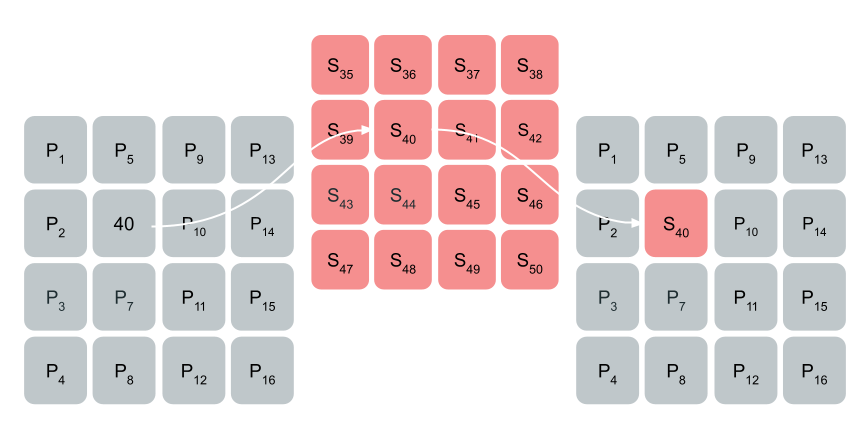Substituting byte “40” in the state with the byte at index 40 in the S-Box

The code for this function could look like the following:

``````def sub_bytes(self, state):
"""AES SubBytes Method.

Args:
state (list): AES State
"""
for i in range(len(state)):
state[i] = self.SBOX[state[i]]
``````

### ShiftRows

The ShiftRows function will rotate each row in the state to the left. The first row is not shifted at all, the second row is shifted by one, the third row by two, and the fourth row by three.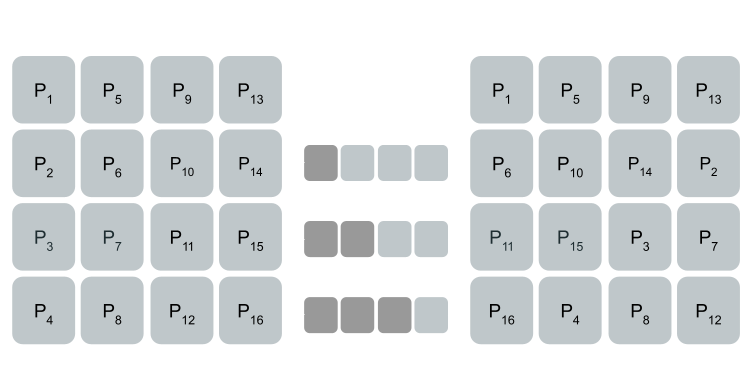AES ShiftRows Function

The code for the ShiftRows function can be:

``````def shift_rows(self, state):
"""AES ShiftRows Method.

Args:
state (list): AES State
"""
for i in range(1, 4):
state[i*4:(i*4)+4] = state[(i*4)+i:(i*4)+4] + state[(i*4):(i*4)+i]
``````

### MixColumns

MixColumns will perform matrix multiplication on each column in the state. The multiplication here also uses the Galois field like in the Key Expansion function. I managed to find a pure Python implementation of this algorithm in GitHub from the user lovasoa which looks like the following:

``````def gmul(a, b):
"""Special AES function used for multiplication

Args:
a (int): Integer to multiply
b (int): Integer to multiply

Returns:
int: The product of the values
"""
p = 0
for c in range(8):
if b & 1:
p ^= a
a <<= 1
if a & 0x100:
a ^= 0x11b
b >>= 1
return p
``````

The Matrix multiplication looks like the following: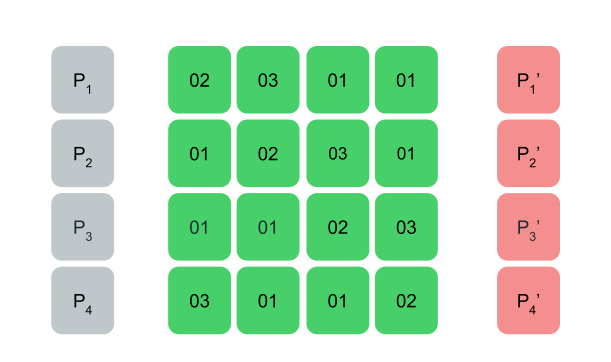AES MixColumns Function

The entire function can be shown as the following Python code:

``````def mix_columns(self, state):
"""AES MixColumns Method.

Args:
state (list): AES State
"""
for i in range(4):
a = state[i]
b = state[i+4]
c = state[i+8]
d = state[i+12]
state[i] = gmul(2, a) ^ gmul(3, b) ^ c ^ d
state[i+4] = a ^ gmul(2, b) ^ gmul(3, c) ^ d
state[i+8] = a ^ b ^ gmul(2, c) ^ gmul(3, d)
state[i+12] = gmul(3, a) ^ b ^ c ^ gmul(2, d)
``````

The AddRoundKey function in AES will XOR part of the expanded key by each column of the state. This can be shown as the following code:

``````def add_round_key(self, state, words):

Args:
state (list): AES State
words (list): List of words from expanded key
"""
for i in range(len(words)):
wb = struct.pack('>I', words[i])
for j in range(4):
state[(j*4)+i] = state[(j*4)+i] ^ wb[j]
``````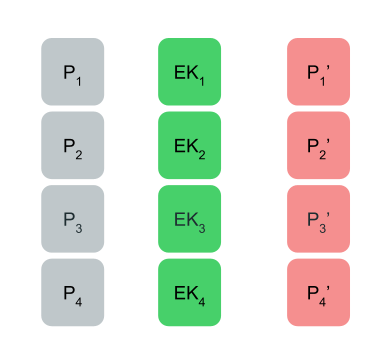AddRoundKey Function

## AES Lookup Table (T-Table) Method

To make the AES algorithm more efficient, the MixColumns, ShiftRows, and SubBytes functions were combined into a a single operation that utilizes five lookup tables. The key expansion algorithm is the same as the original AES algorithm and the state is treated as a normal array and not as a 4x4 matrix.

### Lookup Table Generation

The first four lookup tables (T-Tables) are generated by multiplying each byte of the S-Box by the matrix used in the MixColumns function. This will create four tables containing 256 four-byte words. They can be generated using the following algorithm:

``````T1 = {S[i] * 2, S[i], S[i], S[i] * 3}
T2 = {S[i] * 3, S[i] * 2, S[i], S[i]}
T3 = {S[i], S[i] *  3, S[i] * 2, S[i]}
T4 = {S[i], S[i], S[i] * 3, S[i] * 2}
``````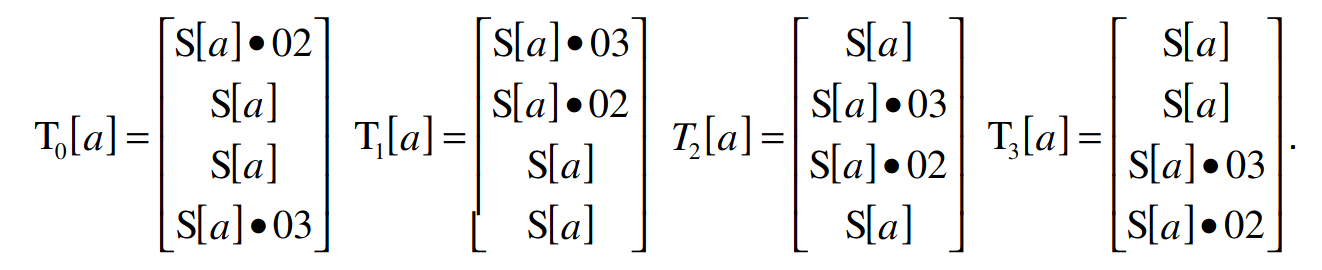AES T-Table Generation

The final T-Table combines each byte of the S-Box into a four-byte word and is only used during the final round of encryption.

``````# Final T-Table generation
T5 = {S[i], S[i], S[i], S[i]}
``````

The entire generation of the lookup tables can look like the following Python code:

``````def generate_t_tables(self):
"""Generates the tables used for the AES lookup table method."""
self.t1 = []
self.t2 = []
self.t3 = []
self.t4 = []
self.t5 = []
for i in range(len(self.SBOX)):
word1 = [gmul(self.SBOX[i], 2), self.SBOX[i],
self.SBOX[i], gmul(self.SBOX[i], 3)]
word2 = [gmul(self.SBOX[i], 3), gmul(self.SBOX[i], 2),
self.SBOX[i], self.SBOX[i]]
word3 = [self.SBOX[i], gmul(self.SBOX[i], 3),
gmul(self.SBOX[i], 2), self.SBOX[i]]
word4 = [self.SBOX[i], self.SBOX[i],
gmul(self.SBOX[i], 3), gmul(self.SBOX[i], 2)]
word5 = [self.SBOX[i]] * 4
self.t1.append(struct.unpack('>I', bytes(word1)))
self.t2.append(struct.unpack('>I', bytes(word2)))
self.t3.append(struct.unpack('>I', bytes(word3)))
self.t4.append(struct.unpack('>I', bytes(word4)))
self.t5.append(struct.unpack('>I', bytes(word5)))
``````

### Round Encryption

For each round of encryption, the AES T-Table algorithm will XOR a byte of the four lookup tables at the index of the original plaintext array. For example, a normal round would look like the following pseudo-code:

``````A = PT[0:4] ^ W
A = PT[4:8] ^ W
A = PT[8:12] ^ W
A = PT[12:16] ^ W

K = 4

for round in NUM_ROUNDS:
S[0:4] = A # Convert word to bytes
S[4:8] = A
S[8:12] = A
S[12:16] = A
A = T1[S] ^ T2[S] ^ T3[S] ^ T4[S] ^ W[K+0]
A = T1[S] ^ T2[S] ^ T3[S] ^ T4[S] ^ W[K+1]
A = T1[S] ^ T2[S] ^ T3[S] ^ T4[S] ^ W[K+2]
A = T1[S] ^ T2[S] ^ T3[S] ^ T4[S] ^ W[K+3]
``````

This method is more efficient than calling the separate SubBytes, ShiftRows, and MixColumns functions because it is all done in one step. There is no need to peform multiple loops or function calls when all the methods are combined at once.

### Final Round

The final round of encryption is similar to the normal rounds except it is using the fifth T-Table and each XOR gets appended to the ciphertext. The following pseudo-code demonstrates this process:

``````CT[0:4] = T5[S] ^ T5[S] ^ T5[S] ^ T5[S] ^ W[K+0]
CT[4:8] = T5[S] ^ T5[S] ^ T5[S] ^ T5[S] ^ W[K+1]
CT[8:12] = T5[S] ^ T5[S] ^ T5[S] ^ T5[S] ^ W[K+2]
CT[12:16] = T5[S] ^ T5[S] ^ T5[S] ^ T5[S] ^ W[K+3]
``````

### AES T-Table Python Code

The full code for the AES T-Table implementation could look like the following:

``````def cipher_t_table(self, pt):
"""Lookup Table AES implementation.

Args:
pt (bytes): Plaintext to encrypt

Returns:
bytes: Encrypted Ciphertext
"""
ct = []
a = [0, 0, 0, 0]
# t is a temporary array to avoid us changing array a while performing the algorithm
t = [0, 0, 0, 0]
a = struct.unpack('>I', pt[0:4]) ^ self.ek
a = struct.unpack('>I', pt[4:8]) ^ self.ek
a = struct.unpack('>I', pt[8:12]) ^ self.ek
a = struct.unpack('>I', pt[12:16]) ^ self.ek
print(a)
for round in range(1, 10):
for i in range(4):
# Using binary rotation instead of splitting words into array
t[i] = (self.t1[(a[i] >> 24) & 0xff] ^
self.t2[(a[(i + 1) % 4] >> 16) & 0xff] ^
self.t3[(a[(i + 2) % 4] >> 8) & 0xff] ^
self.t4[(a[(i + 3) % 4]) & 0xff] ^
self.ek[(round*4)+i])
a = t.copy()

# Final round of encryption
for i in range(4):
ct.append(self.SBOX[(a[i] >> 24) & 0xff] ^
((self.ek[-4+i] >> 24) & 0xff))
ct.append(self.SBOX[(a[(i + 1) % 4] >> 16) & 0xff] ^
((self.ek[-4+i] >> 16) & 0xff))
ct.append(self.SBOX[(a[(i + 2) % 4] >> 8) & 0xff] ^
((self.ek[-4+i] >> 8) & 0xff))
ct.append(self.SBOX[(a[(i + 3) % 4]) & 0xff] ^
((self.ek[-4+i]) & 0xff))
return bytes(ct)
``````

## Identifying AES in Assembly Code

### Identifying the S-Box/T-Tables

A quick win when trying to identify if a binary is using AES is to look for either the Substitution Box or any of the lookup tables from the T-Tables implementation. For example, in my previous post on sodinokibi ransomware, it was found that the sample was utilizing AES to encrypt data. We can search for the first word of one of the T-Tables in Cutter and see that it exists in the binary.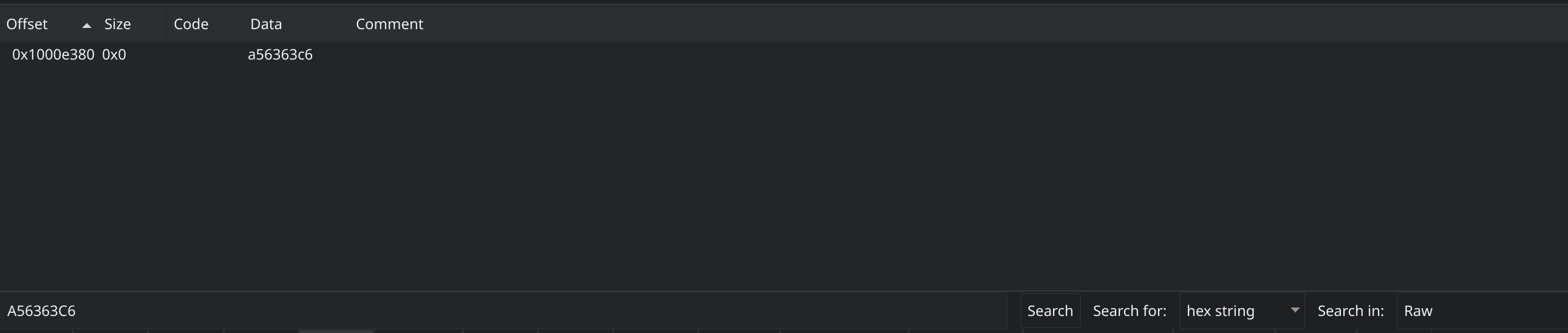Searching for word in T-Table in Cutter

Now if we go to that location we can confirm that this is one of the T-Tables used by AES.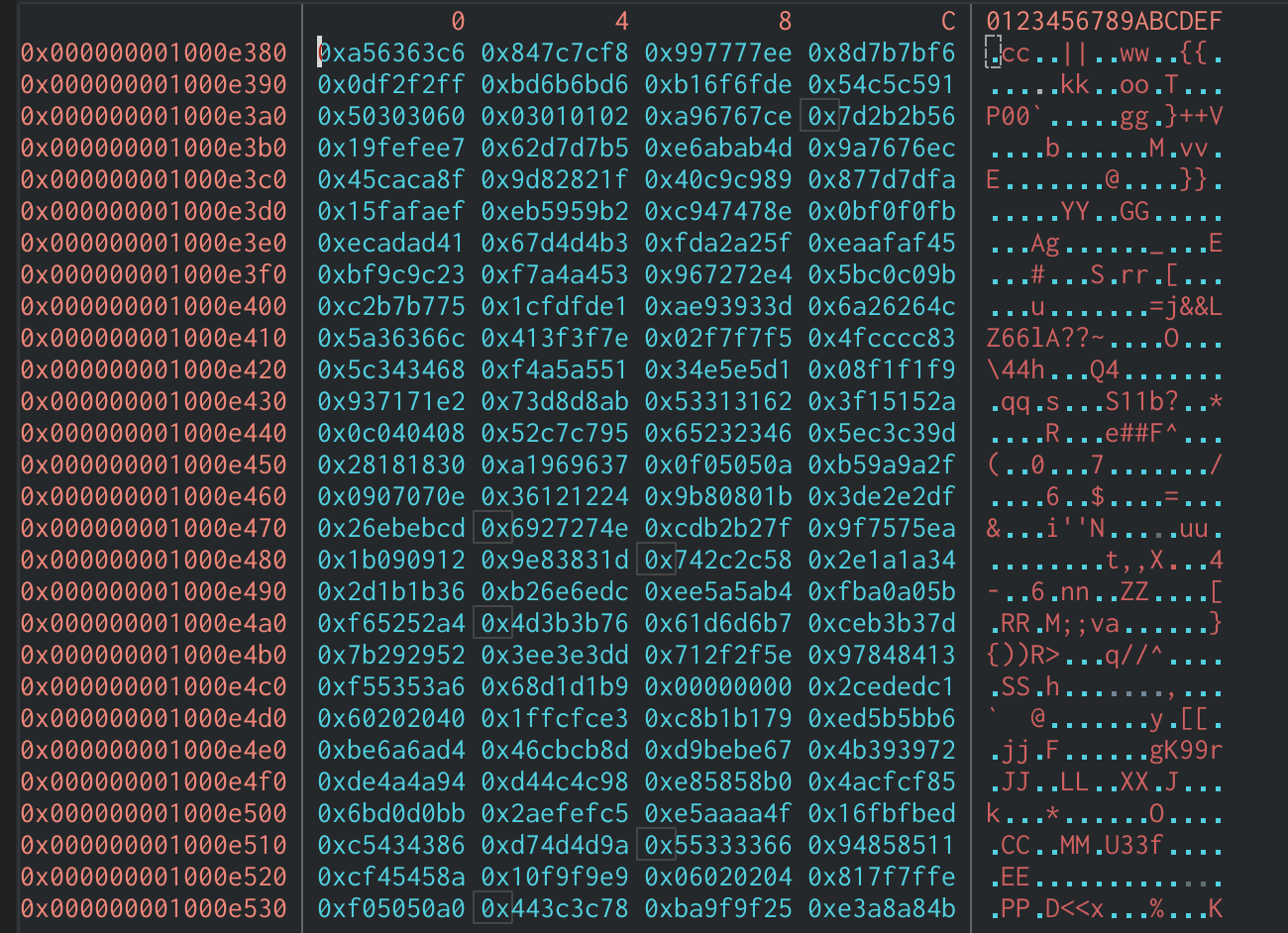Hexdump of T-Table in Cutter

This is a great indicator to identify AES. If we search for references to this array in Cutter, we will see one of the XOR operations during a round of encryption which confirms the use of AES.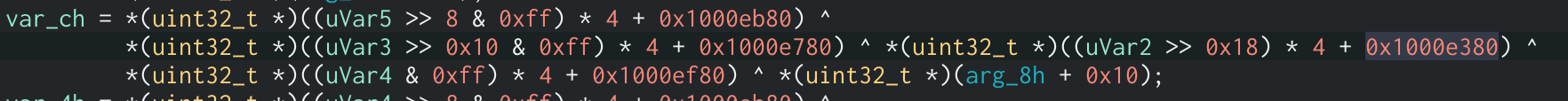T-Table XOR Assembly Code

### Identifying Normal AES Functions

Another way to tell if AES is being used is to identify the individual ShiftRows, SubBytes, MixColumns, and AddRoundKey functions. Breaking down and identifying each function individually is more efficient than reverse engineering a large amount of code at once. We will be using the GitHub project tiny-AES, a pure implementation of the AES algorithm using C code, as an example to show how the code would look in Assembly.

The AddRoundKey function in this binary takes three arguments. The first argument is shifted left by four then AND’d by `0xff0` which is the same as multiplying it by 16, the same size as the state. This is a good indicator that this argument will hold the current round number. This value is then added to the third argument of the function. Because the state cannot be more than 16 bytes in length, we can assume that the third argument is the expanded key and the second argument is the state. After this, we can see two loops that will XOR the state column-by-column against the expanded key.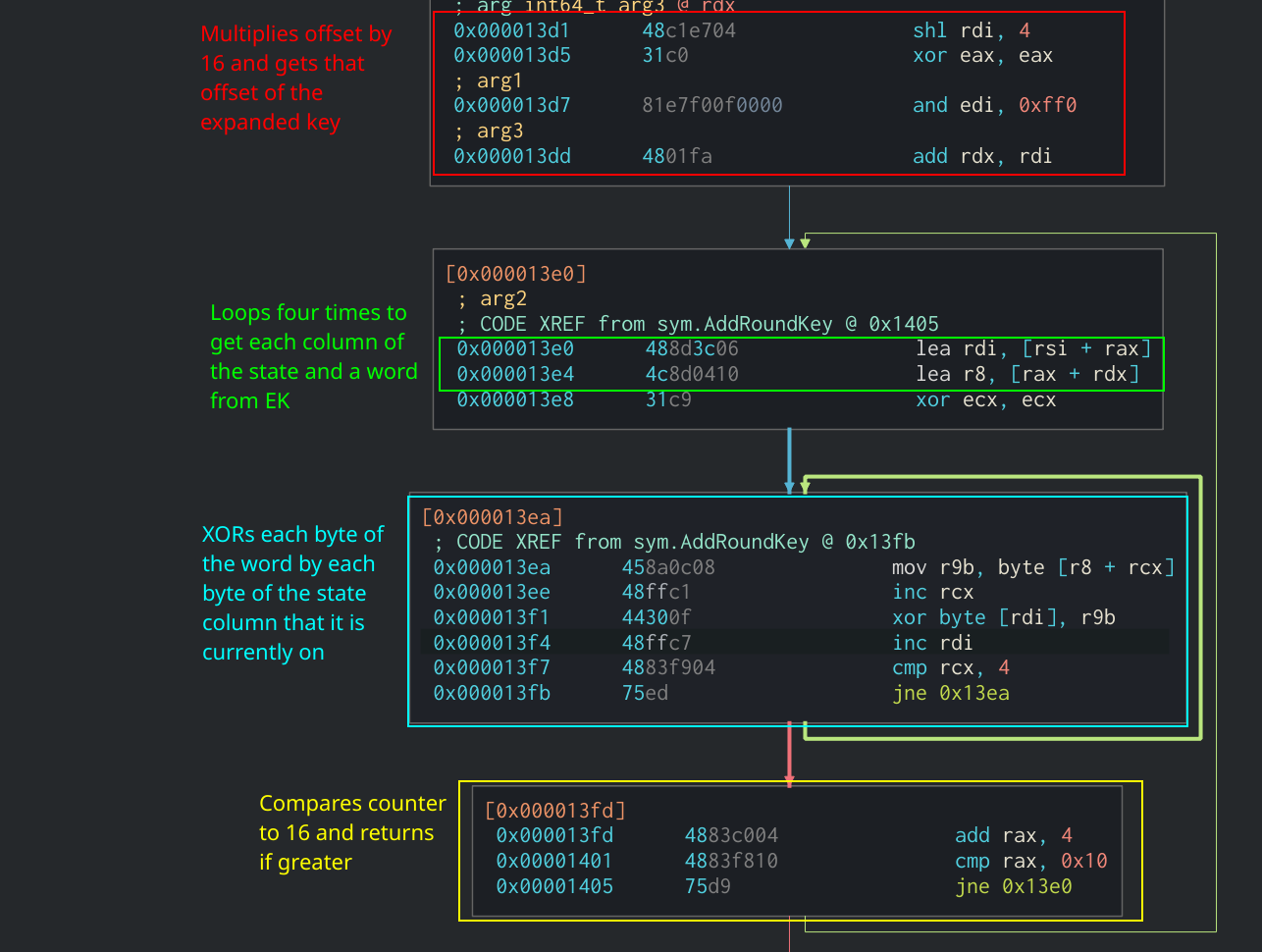AddRoundKey Function in Assembly

#### SubBytes in Assembly

When compiling the code from the GitHub project, the SubBytes is added in-line in the main cipher function rather than making a function call. We are able to identify it as SubBytes by the fact that it takes each byte of the state and uses it as an offset in the S-Box array. Then it will take the byte at that offest and put it into the state at the current index. We can see all of this being done in two loops in the Cipher function: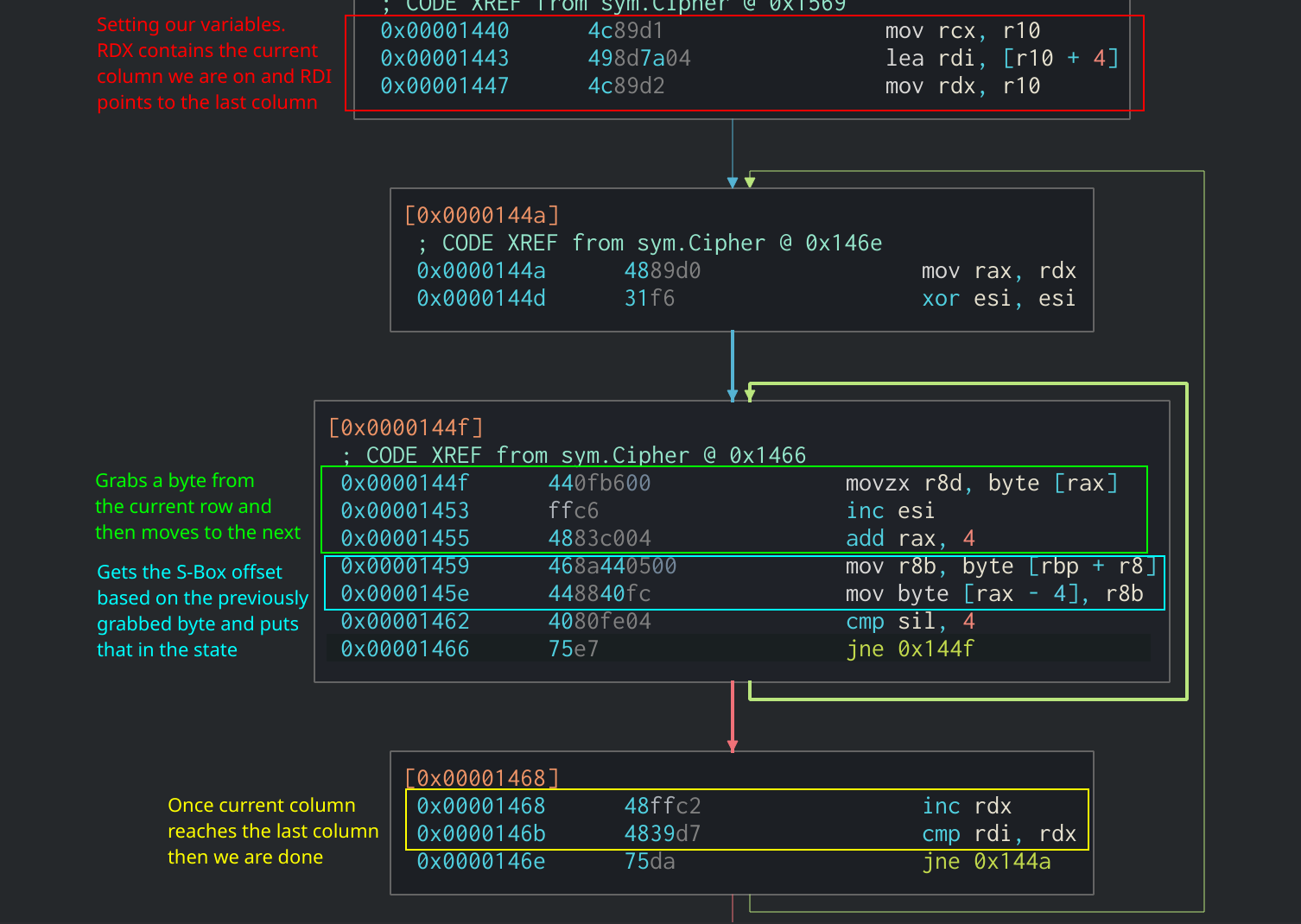SubBytes function in Assembly

#### ShiftRows in Assembly

The ShiftRows function is also added in-line in the main cipher function. It is pretty easy to spot as it consists of several `mov` instructions on the state to shift each row the correct amount of times.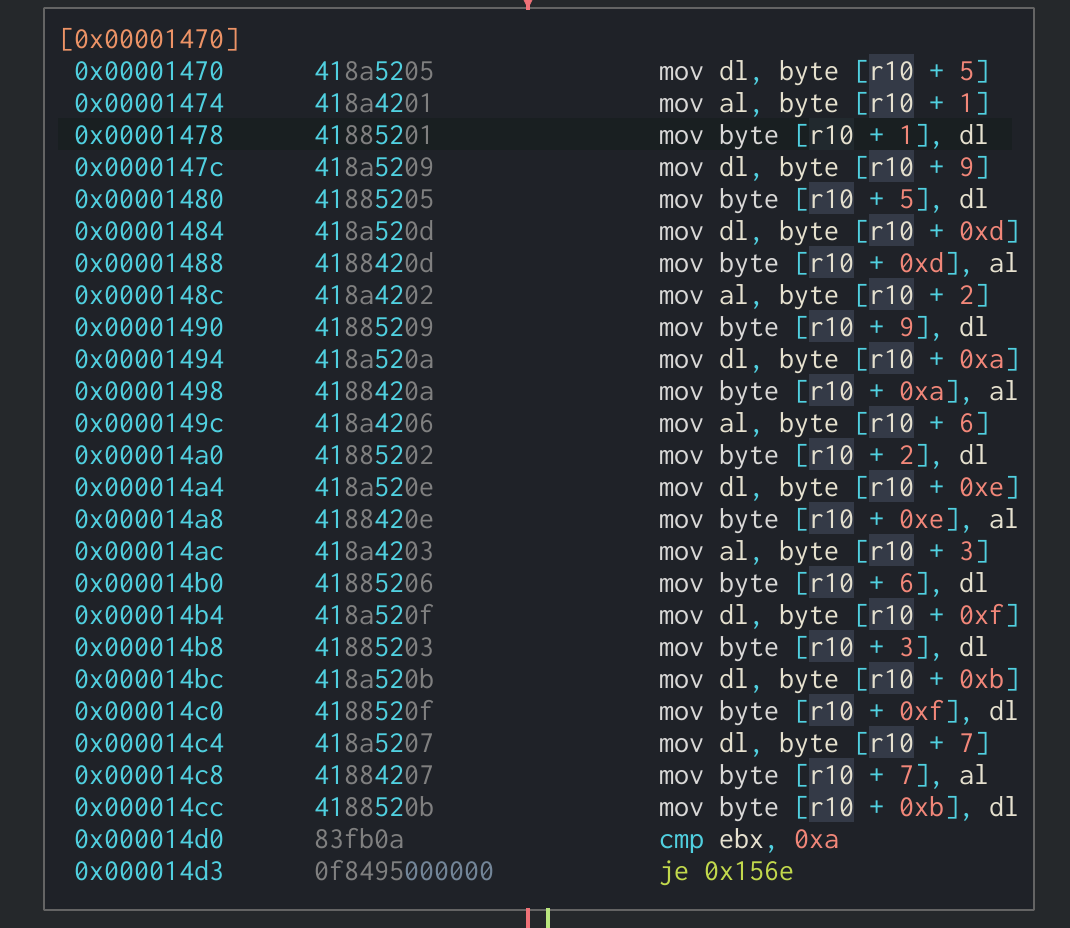ShiftRows function in Assembly

#### MixColumns in Assembly

Finally, the MixColumns function is also in-line. It can be identified through multiple XOR operations being done on the state along with the Galois Field multiplication function. The results of the XOR operations are then put back into the state. In this binary, the MixColumns function will loop four times and perform the XOR operations on each row’s values then update that row with the new values. This can all be seen in the following section: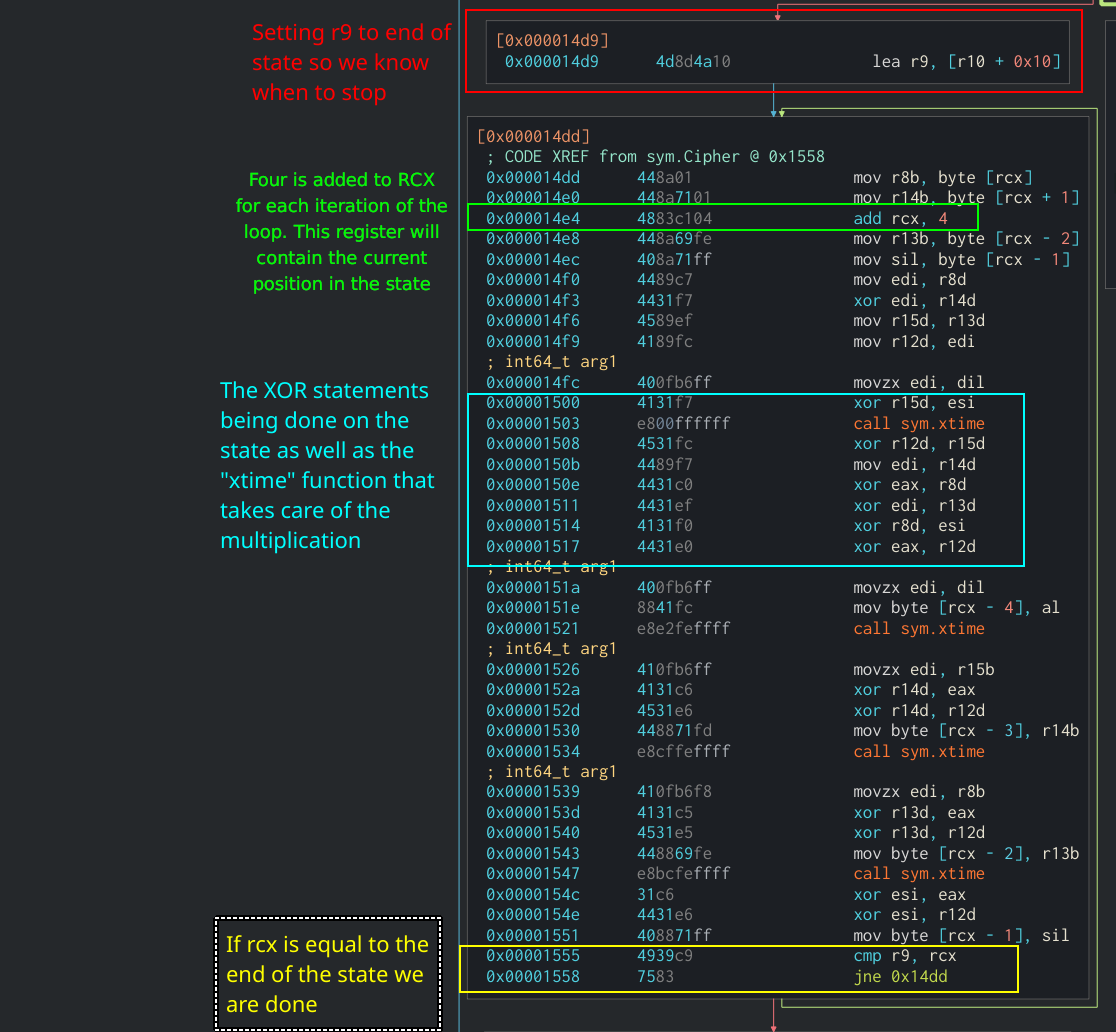MixColumns in Assembly

### Identifying Key Expansion

Since the Key Expansion algorithm is the same between the T-Table and normal implementations of AES, this indicator can be used to identify AES in other binaries. The first part of the Key Expansion algorithm copies the first four words of the original key into the expanded key. In Assembly, this would look like several `mov` instructions moving a byte of the original key into the new expanded key. This example is from the tiny-AES GitHub project mentioned earlier: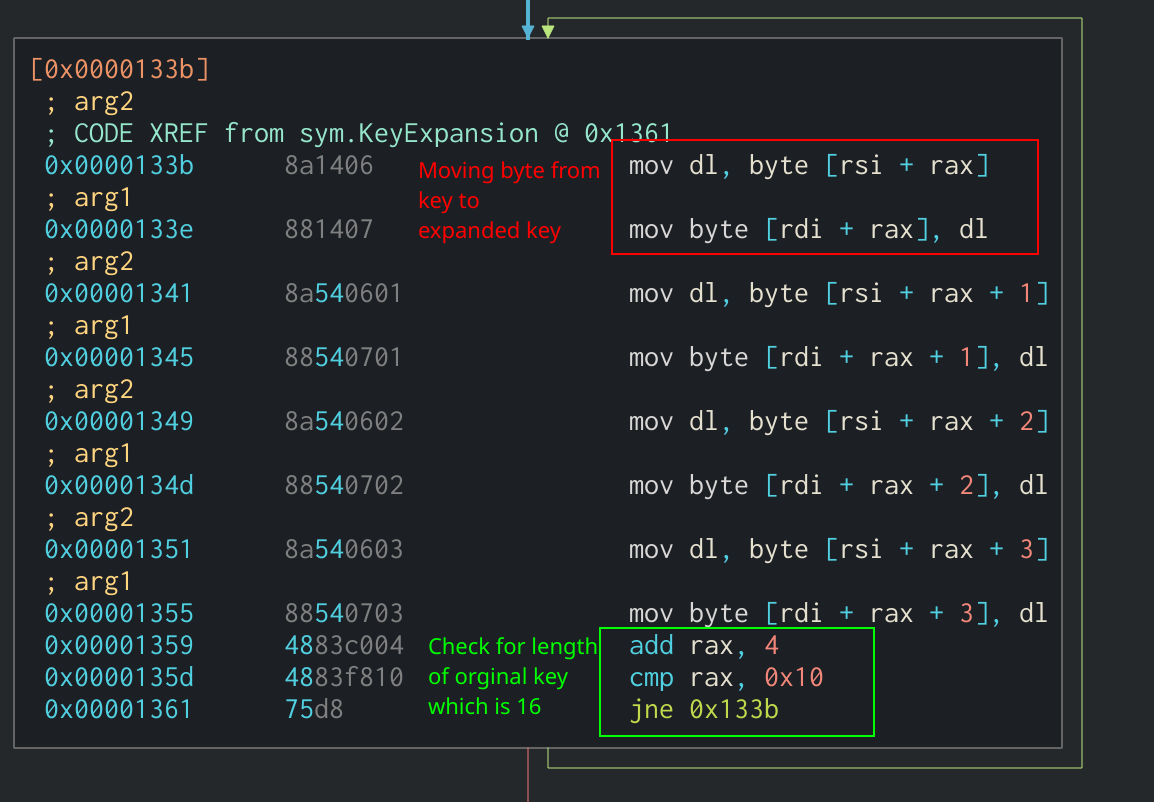First part of Key Expansion algorithm in Assembly

The next part of the Key Expansion algorithm checks to see if the current index is divisible by four. If it is, the application will split the previous word into separate registers and performs a lookup of each one in the S-Box (`r9`). Afterwards, it rotates each byte individually to a new register. This is an in-line implementation of the SubWord and RotWord functions that were described earlier in the article. Finally, the most significant byte of the new word is XOR’d by one of the Round Constants stored in `r10`.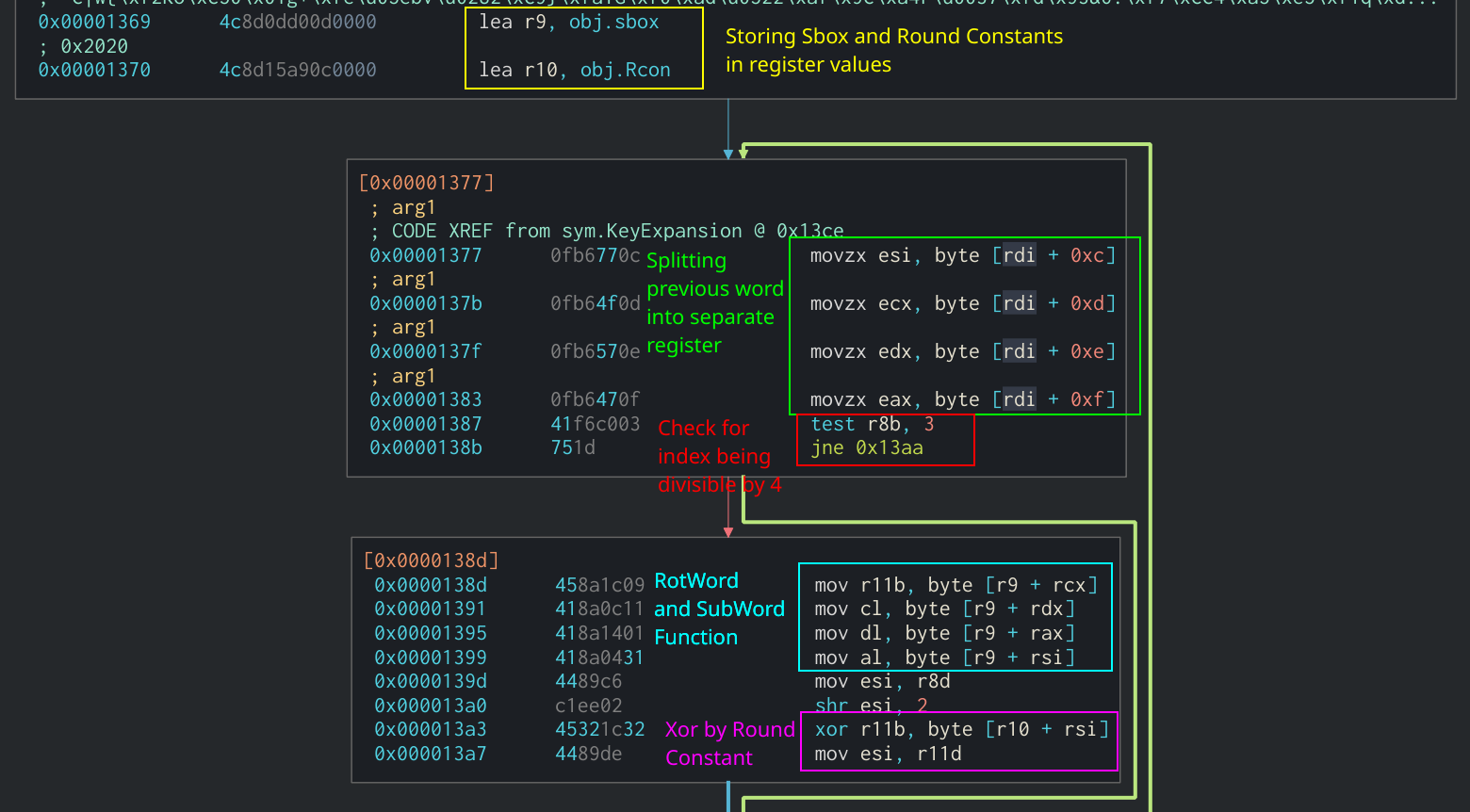RotWord, SubWord, and Round Constant use in Assembly

We can see in the Assembly that the new word is being XOR’d by the word four indices previous in the expanded key.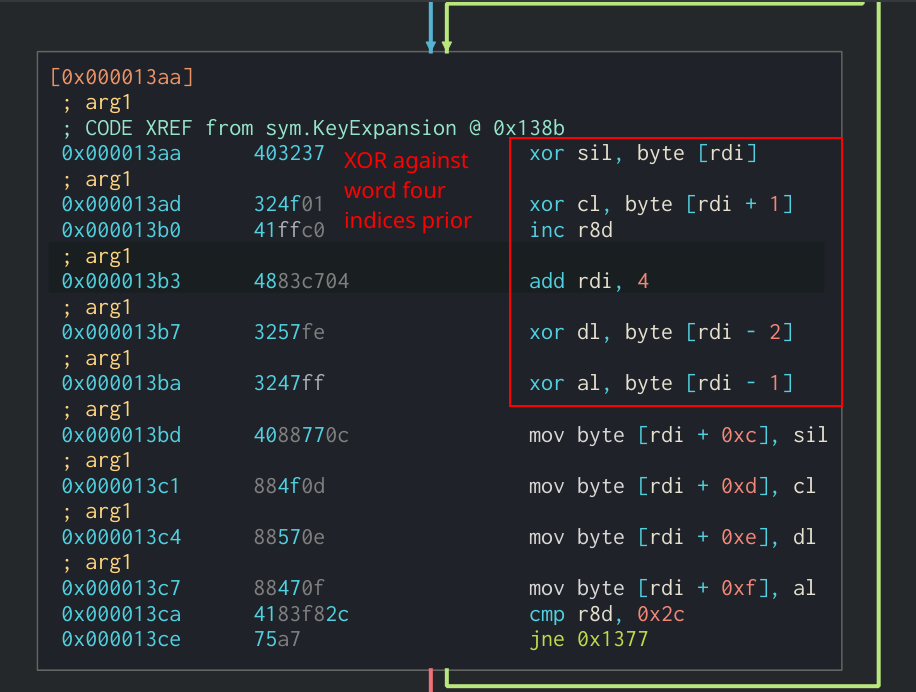Final section of Key Expansion algorithm

## Conclusion

Hopefully this article can help analysts identify implementations of the AES algorithm in other binaries. For additional reading into some of the design decisions for AES and more info on the mathematics behind it, I recommend reading two of the official NIST documentations on AES: